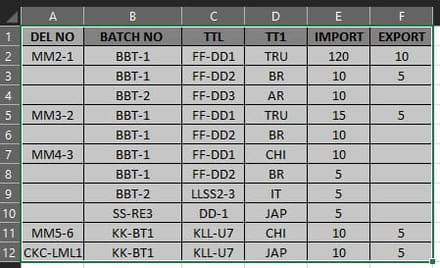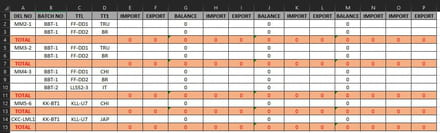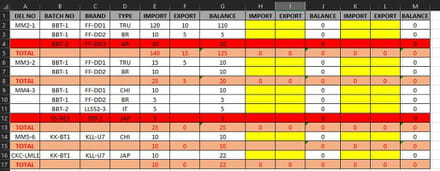# Add missing data before TOTAL row after matching between two sheets

[Solved]
Posts
3
Registration date
Friday October 1, 2021
Status
Member
Last seen
October 15, 2021
-
Posts
3
Registration date
Friday October 1, 2021
Status
Member
Last seen
October 15, 2021
-
Hello,

I'm new begginer in vba and I hope some help
I have data in two sheets . should match data beween them. the match depend in COL A,B,C,D .each item in COL A contain data in COL B,C,D .
the item in COL A start from first cell contains item and finish to last empty cell before start new item .
as you see how the data were in sheet before ,and after match should add new data based on COL A between two sheets when there are new items in COL B,C,D for the item in COL A in sheet DATA but not existed in sheet before then should add it before TOTAL row with the same formatting and borders also formulas in sheet before under that item .
so the result should show in sheet before as I put in sheet after
important note : when match data and are existed in two sheets then should pull the values as highlighted by yellow cells in columns IMPORT,EXPORT but should be to last empty columns (IMPORT,EXPORT) because every time i will insert three columns the same thing about the new data added before row TOTAL . should be to last empty columns import, export.
the data increases every time in two sheets

sheet DATAsheet BEFOREsheet AFTERwith considering the row TOTAL has many formula should not affect when add new data

thanks

## 2 replies

Posts
2817
Registration date
Sunday September 12, 2010
Status
Moderator
Last seen
October 14, 2021
486
Hi Mussa,

Give the following code a try and let us know how it performs:
```Sub RunMe()
Dim mFind As Range
Dim sString, dString, sValue As String
Dim dExist As Boolean
Dim dRow, uRow As Long

Sheets("DATA").Select
With Sheets("REPORT")
For Each cell In Range("B2:B" & Range("B" & Rows.Count).End(xlUp).Row)
If cell.Offset(0, -1).Value = vbNullString Then
sString = cell.Offset(0, -1).End(xlUp).Value & cell.Value & cell.Offset(0, 1).Value & cell.Offset(0, 2).Value
Else
sString = cell.Offset(0, -1).Value & cell.Value & cell.Offset(0, 1).Value & cell.Offset(0, 2).Value
End If
dExist = False
Set mFind = .Columns("B").Find(cell.Value)
If Not mFind Is Nothing Then
Do
If mFind.Offset(0, -1).Value = vbNullString Then
dString = mFind.Offset(0, -1).End(xlUp).Value & mFind.Value & mFind.Offset(0, 1).Value & mFind.Offset(0, 2).Value
Else
dString = mFind.Offset(0, -1).Value & mFind.Value & mFind.Offset(0, 1).Value & mFind.Offset(0, 2).Value
End If
If sString = dString Then
.Cells(mFind.Row, Columns.Count).End(xlToLeft).Offset(0, -2).Value = cell.Offset(0, 3).Value
.Cells(mFind.Row, Columns.Count).End(xlToLeft).Offset(0, -1).Value = cell.Offset(0, 4).Value
dExist = True
End If
Set mFind = .Columns("B").FindNext(mFind)
End If
If dExist = False Then
If cell.Offset(0, -1).Value = vbNullString Then
sValue = cell.Offset(0, -1).End(xlUp).Value
Else
sValue = cell.Offset(0, -1).Value
End If
Set mFind = .Columns("A").Find(sValue)
If Not mFind Is Nothing Then
If mFind.Offset(1, 0).Value = vbNullString Then
dRow = mFind.End(xlDown).Row
Else
dRow = mFind.Offset(1, 0).Row
End If
.Rows(dRow - 1).Copy
.Rows(dRow).Insert
.Rows(dRow).SpecialCells(xlCellTypeConstants).ClearContents
.Range("B" & dRow).Value = cell.Value
.Range("C" & dRow).Value = cell.Offset(0, 1).Value
.Range("D" & dRow).Value = cell.Offset(0, 2).Value
uRow = .Cells(dRow, "A").End(xlUp).Row
.Cells(dRow + 1, 5).Formula = "=SUM(" & Range(Cells(uRow, 5), Cells(dRow, 5)).Address(False, False) & ")"
.Cells(dRow + 1, 5).AutoFill Destination:=.Range(.Cells(dRow + 1, 5), .Cells(dRow + 1, .Cells(dRow + 1, Columns.Count).End(xlToLeft).Column))
Else
dRow = .Range("A" & Rows.Count).End(xlUp).Offset(1, 0).Row
.Rows(dRow - 2 & ":" & dRow - 1).Copy .Rows(dRow)
.Rows(dRow).SpecialCells(xlCellTypeConstants).ClearContents
.Range("A" & dRow).Value = sValue
.Range("B" & dRow).Value = cell.Value
.Range("C" & dRow).Value = cell.Offset(0, 1).Value
.Range("D" & dRow).Value = cell.Offset(0, 2).Value
End If
With .Cells(dRow, Columns.Count).End(xlToLeft)
.Offset(0, -2).Value = cell.Offset(0, 3).Value
.Offset(0, -1).Value = cell.Offset(0, 4).Value
End With
End If
Next cell
End With
End Sub```

Best regards,
Trowa
Posts
3
Registration date
Friday October 1, 2021
Status
Member
Last seen
October 15, 2021

Hi Trowa,
it must hard work,much time to do that .
that's very excellent ! I tested and works without any problem
thanks very much for your great assistance .
Posts
2817
Registration date
Sunday September 12, 2010
Status
Moderator
Last seen
October 14, 2021
486
Hi Mussa,

Question about the important note: When data is matched between the 2 sheets, you want to put the numbers in the last free import/export columns. Then how do you prevent numbers from being repeated?

Example:
The combined values of col A, B, C and D of row 2: MM2-1, BBT-1,FF-DD1,TRU, can also be found on the second sheet. The numbers are put in col E and F, but upon rerunning the code, the numbers will also be put in col K and L, as they are the last free import/export columns at the moment.

To me this seems as an unwanted result. How do you envision to bypass this dilemma?

Best regards,
Trowa
Posts
3
Registration date
Friday October 1, 2021
Status
Member
Last seen
October 15, 2021

Hi Trowa,

about repeating the value in last columns in IMPORT, EXPORT don't worry about it .
because the values will change every week . so the data in first sheet depends on another file .
in other meaning will pull the data from weekly file to sheet DATA ,then the data changes whether add new data or change in value . that's why I want when run macro repeatedly should fill in last empty columns IMPORT,EXPORT .
last thing as you see in third picture this is just to understand my requirements . the matching is between two sheets(DATA,REPORT) the result should show in sheet REPORT
I hope to did not forget another thing until does not happen any confusing .